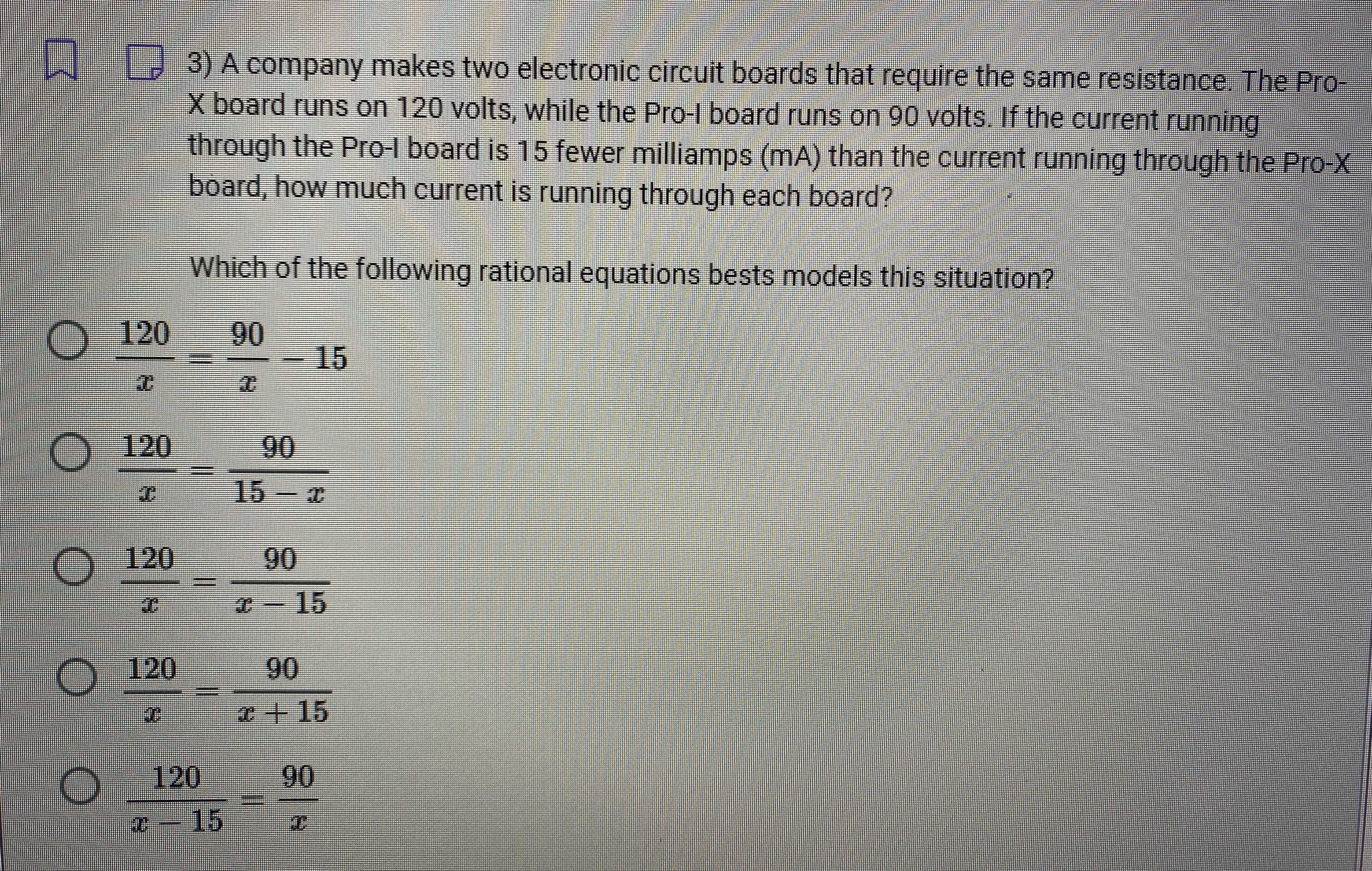### Still have math questions?

Algebra
Question3) A company makes two electronic circuit boards that require the same resistance. The Pro- X board runs on $$120$$ volts, while the Pro-I board runs on $$90$$ volts. If the current running through the Pro-I board is $$15$$ fewer milliamps (mA) than the current running through the Pro- $$X$$ board, how much current is running through each board?

Which of the following rational equations bests models this situation?

$$\frac { 120 } { x } = \frac { 90 } { x } - 15$$

$$\frac { 120 } { x } = \frac { 90 } { 15 - x }$$

$$\frac { 120 } { x } = \frac { 90 } { x - 15 }$$

$$\frac { 120 } { x } = \frac { 90 } { x + 15 }$$

$$\frac { 120 } { x - 15 } = \frac { 90 } { x }$$